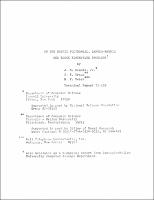## On the Matrix Polynomial, Lamda-Matrix and Block Eigenvalue Problems

 dc.contributor.author Dennis, John E., Jr. en_US dc.contributor.author Traub, J.F. en_US dc.contributor.author Weber, R.P. en_US dc.date.accessioned 2007-04-19T17:56:16Z dc.date.available 2007-04-19T17:56:16Z dc.date.issued 1971-09 en_US dc.description.abstract A matrix $S$ is a solvent of the matrix polynomial $M(X) \equiv X^{m} + A_{1}X^{m-1} + \cdots + A_{m}$, if $M(S) = \stackrel{0}{=}$, where $A_{1}, X$ and $S$ are square matrices. We present some new mathematical results for matrix polynomials, as well as a globally convergent algorithm for calculating such solvents. In the theoretical part of this paper, existence theorems for solvents, a generalized division, interpolation, a block Vandermonde, and a generalized Lagrangian basis are studied. Algorithms are presented which generalize Traub's scalar polynomial methods, Bernoulli's method, and eigenvector powering. The related lambda-matrix problem, that of finding a scalar $\lambda$ such that $I \lambda^{m} + A_{1} \lambda^{m-1} + \cdots + A_{m}$ is singular, is examined along with the matrix polynomial problem. The matrix polynomial problem can be cast into a block eigenvalue formulation as follows. Given a matrix $A$ of order mn, find a matrix $X$ of order n, such that $AV=VX$, where $V$ is a matrix of full rank. Some of the implications of this new block eigenvalue formulation are considered. en_US dc.format.extent 4207450 bytes dc.format.extent 3115144 bytes dc.format.mimetype application/pdf dc.format.mimetype application/postscript dc.identifier.citation http://techreports.library.cornell.edu:8081/Dienst/UI/1.0/Display/cul.cs/TR71-109 en_US dc.identifier.uri https://hdl.handle.net/1813/5954 dc.language.iso en_US en_US dc.publisher Cornell University en_US dc.subject computer science en_US dc.subject technical report en_US dc.title On the Matrix Polynomial, Lamda-Matrix and Block Eigenvalue Problems en_US dc.type technical report en_US
##### Original bundle
Now showing 1 - 2 of 2Name:
71-109.pdf
Size:
4.01 MB
Format: